## Separate octets of IP address in Excel

Have you ever tried to split the IP addresses into separate columns in Excel worksheet? Maybe the Text to Column feature can help you to solve this job quickly, but, this article, I will talk about some formulas for achieving this task in Excel.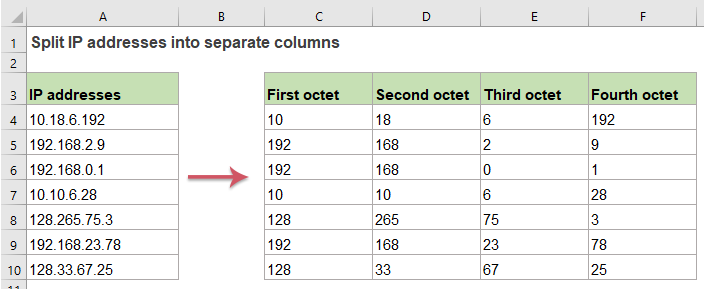#### Split octets of IP addresses into separate columns in Excel

Normally, in Excel, the LEFT, MID, LEN and FIND functions can help you to extract each octet from the IP address into different columns. Please do with the following steps:

##### Split the first octet of the IP addresses:

To extract the first octet of the IP address, please apply the below formula into a blank cell:

=LEFT(A2,FIND(".",A2)-1)

And then, drag the fill handle down to the cells that you want to apply this formula, and all the first octet of the IP addresses have been extracted, see screenshot: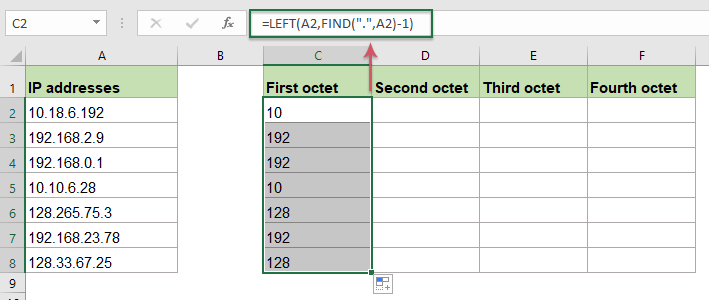##### Explanation of the formula:

FIND(".",A2)-1: This FIND function returns the position of the first dot from cell A2, subtracts 1 means to exclude the dot itself, you will get the result 2.

LEFT(A2,FIND(".",A2)-1)=LEFT(A2, 2):The LEFT function is used to extract 2 characters from the left side of the string in cell A2.

##### Split the second octet of the IP addresses:

For getting the second octet of the IP addresses, please copy or enter the following formula into a blank cell:

=MID(A2,FIND(".",A2)+1,FIND(".",A2,FIND(".",A2)+1)-FIND(".",A2)-1)

Then, drag the fill handle down to the cells to fill this formula, ad you will get the result as you need, see screenshot: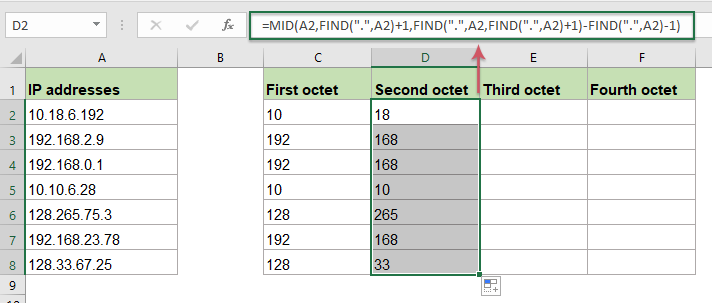##### Explanation of the formula:

1. FIND(".",A2)+1: This FIND function returns the position of the first dot from cell A2, adds 1 means to the extraction from the next character, you will get the result the number 4. This part of formula is recognized as the start_num argument of the MID function.

2. FIND(".",A2,FIND(".",A2)+1)-FIND(".",A2)-1: This part is recognized as the num_chars argument within the MID function.

• FIND(".",A2,FIND(".",A2)+1)= FIND(".",A2,4): This FIND function will return the position of the second dot. And you will get the number 6.
• FIND(".",A2,FIND(".",A2)+1)-FIND(".",A2)-1=6-3-1: Subtract the position of the first dot from the position of the second dot, and then subtract 1 from the result means to remove a leading dot. And the result is 2.

3. MID(A2,FIND(".",A2)+1,FIND(".",A2,FIND(".",A2)+1)-FIND(".",A2)-1)=MID(A2, 4, 2): Finally, this MID function is used to extract 2 characters which start at the fourth character from cell A2.

##### Split the third octet of the IP addresses:

To extract the third octet of the IP addresses, the MID and FIND functions also can do you a favor, please enter or copy the following formula into a blank cell:

=MID(A2,FIND(".",A2,FIND(".",A2)+1)+1,FIND(".",A2,FIND(".",A2,FIND(".",A2)+1)+1)-(FIND(".",A2,FIND(".",A2)+1)+1))

Then, drag the fill handle down to get the results as you need, and only the third octet of the IP addresses have been split out, see screenshot: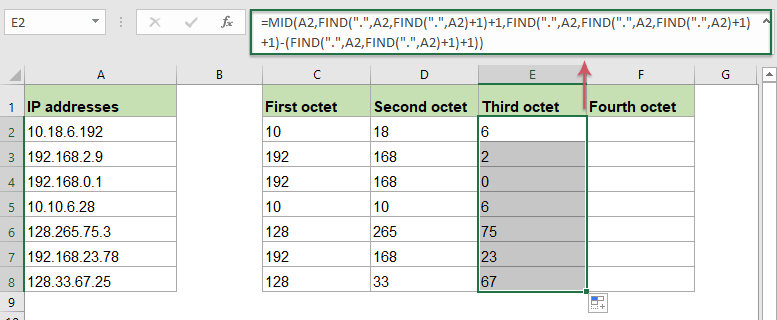##### Explanation of the formula:

1. FIND(".",A2,FIND(".",A2)+1)+1: This nested FIND function is used to get the position of the second dot in cell A2, adding 1 means to the extraction from the next character, and you will get the number 7. This part is recognized as the start_num argument of the MID function.

2. FIND(".",A2,FIND(".",A2,FIND(".",A2)+1)+1)-(FIND(".",A2,FIND(".",A2)+1)+1): This part of formula is recognized as the num_chars argument of the MID function.

• FIND(".",A2,FIND(".",A2,FIND(".",A2)+1)+1)=FIND(".",A2, 7): This nested FIND function is used to get the position of the third dot in cell A2, it returns the number 8.
• FIND(".",A2,FIND(".",A2,FIND(".",A2)+1)+1)-(FIND(".",A2,FIND(".",A2)+1)+1)=8-7: Subtract the position of the second dot from the position of the third dot, And the result is 1.

3.MID(A2,FIND(".",A2,FIND(".",A2)+1)+1,FIND(".",A2,FIND(".",A2,FIND(".",A2)+1)+1)-(FIND(".",A2,FIND(".",A2)+1)+1))=MID(A2, 7, 1): This MID function will get 1 character which start at the seventh character from cell A2.

##### Split the fourth octet of the IP addresses:

The below formula can help to extract the last octet of the IP address, please copy or enter the formula into a blank cell:

=MID(A2,FIND(".",A2,FIND(".",A2,FIND(".",A2)+1)+1)+1,LEN(A2)-FIND(".",A2,FIND(".",A2,FIND(".",A2)+1)+1))

And then, drag the fill handle to fill this formula to other cells you need, you will get the result as below screenshot shown: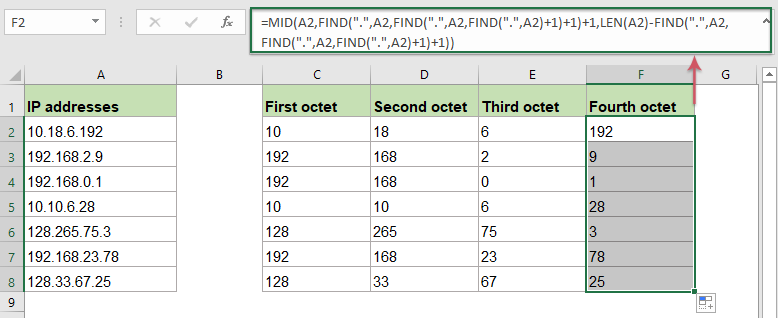##### Explanation of the formula:

1. FIND(".",A2,FIND(".",A2,FIND(".",A2)+1)+1)+1: This nested FIND function is used to get the position of the third dot in cell A2, adding 1 means to the extraction from the next character, and you will get the number 9. This part is recognized as the start_num argument of the MID function.

2. LEN(A2)-FIND(".",A2,FIND(".",A2,FIND(".",A2)+1)+1):

• LEN(A2): The LEN function returns the total number of the characters in cell A2. It will get the number 11.
• LEN(A2)-FIND(".",A2,FIND(".",A2,FIND(".",A2)+1)+1)=11-8: The total length of cell A2 subtracts the position of the third dot to get the number of the remaining character. This will get the number 3.

3. MID(A2,FIND(".",A2,FIND(".",A2,FIND(".",A2)+1)+1)+1,LEN(A2)-FIND(".",A2,FIND(".",A2,FIND(".",A2)+1)+1))=MID(A2, 9, 3): At last, this MID function will get 3 character which start at the ninth character from cell A2.

#### Relative functions used:

• LEFT:
• The LEFT function extracts the given number of characters from the left side of a supplied string.
• MID:
• The MID function is used to find and return a specific number of characters from the middle of given text string.
• FIND:
• The FIND function is used to find a string within another string, and returns the starting position of the string inside another one.
• LEN:
• The LEN function returns the number of characters in a text string.

#### More articles:

• Split Text And Numbers In A Cell In Excel
• Supposing a cell data is mixed with text and numbers, how can you split them into separate column cells? This tutorial is going to show you the detailed steps to get it down with formulas.

### The Best Office Productivity Tools

#### Kutools for Excel - Helps You To Stand Out From Crowd

 Popular Features: Find, Highlight or Identify Duplicates  |  Delete Blank Rows  |  Combine Columns or Cells without Losing Data  |  Round without Formula ... Super VLookup: Multiple Criteria  |  Multiple Value  |  Across Multi-Sheets  |  Fuzzy Lookup... Adv. Drop-down List: Easy Drop Down List  |  Dependent Drop Down List  |  Multi-select Drop Down List... Column Manager: Add a Specific Number of Columns  |  Move Columns  |  Toggle Visibility Status of Hidden Columns  |  Compare Columns to Select Same & Different Cells ... Featured Features: Grid Focus  |  Design View  |  Big Formula Bar  |  Workbook & Sheet Manager | Resource Library (Auto Text)  |  Date Picker  |  Combine Worksheets  |  Encrypt/Decrypt Cells  |  Send Emails by List  |  Super Filter  |  Special Filter (filter bold/italic/strikethrough...) ... Top 15 Toolsets:  12 Text Tools (Add Text, Remove Characters ...)  |  50+ Chart Types (Gantt Chart ...)  |  40+ Practical Formulas (Calculate age based on birthday ...)  |  19 Insertion Tools (Insert QR Code, Insert Picture from Path ...)  |  12 Conversion Tools (Numbers to Words, Currency Conversion ...)  |  7 Merge & Split Tools (Advanced Combine Rows, Split Excel Cells ...)  |  ... and more

Kutools for Excel Boasts Over 300 Features, Ensuring That What You Need is Just A Click Away...#### Office Tab - Enable Tabbed Reading and Editing in Microsoft Office (include Excel)

• One second to switch between dozens of open documents!
• Reduce hundreds of mouse clicks for you every day, say goodbye to mouse hand.
• Increases your productivity by 50% when viewing and editing multiple documents.
• Brings Efficient Tabs to Office (include Excel), Just Like Chrome, Edge and Firefox.